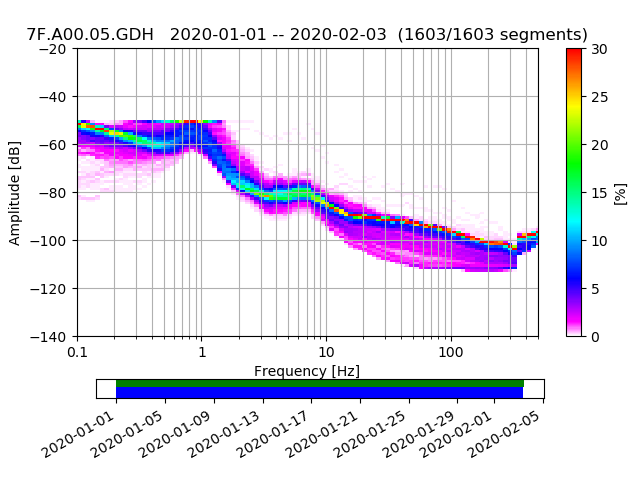# Y axis limits in PPSD plots

How can I change the default y-axis limits in PPSD.plot?

Thanks, Rob

Use show=False, don't use filename=... and then work with the returned
matplotlib Figure object and call pyplot show when done or save to file.

Something like
fig = ppsd.plot(...)
fig.axes.set_ylim(..., ...)

cheers,
Tobias

Thanks for the help. It's not quite working, though...

I use the following code:

fig = ppsd.plot(show=False,xaxis_frequency=True,period_lim=(0.002, 10),cmap=pqlx,show_noise_models=False)
fig.axes.set_ylim(-140, -20)

And get, for example, the attached figure. Although I can change the upper y-axis limit to -20, the plot doesn't show anything above -50, which is the upper y-axis limit in the default settings, even though there is signal up there. Note that I am plotting hydrophone data, which is why the dB levels are not aligned with 'normal' seismometer data.

Does this have something to do with db_bin_edges setting in the ppsd routine?

Thanks, Rob

Quoting Tobias Megies <megies@geophysik.uni-muenchen.de>:So you do not want the plot limits to change, but the processing
parameters. You need to specify that when initializing your PPSD before
feeding in data:

http://docs.obspy.org/master/packages/autogen/obspy.signal.spectral_estimation.PPSD.init.html?highlight=db_bins

ppsd = PPSD(..., db_bins=...)

best,
Tobias

Thanks. I now see it has to be done by setting the db_bins parameter.

Best, Rob

Quoting Tobias Megies <megies@geophysik.uni-muenchen.de>: